StatLect

# Indicator function

The indicator function of an event is a random variable that takes:

• value 1 when the event happens;

• value 0 when the event does not happen.

Indicator functions are also called indicator random variables.## Things to remember

To understand the following definition, you need to remember that a random variableis a function:

• from the sample space(the set of all possible random outcomes)

• to the set of real numbers.

Ifis one of the possible outcomes, thenis the value taken bywhen the realized outcome is.

Also remember that an eventis a subset of the sample space.

## Definition

Here is the definition.

Definition Letbe a sample space andbe an event. The indicator function of, denoted by, is the random variable defined as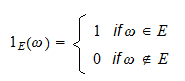Sometimes we also use the notationwhereis the Greek letter Chi.

## Example

We toss a die and one of the six numbers from 1 to 6 can appear face up.

The sample space isDefine the eventdescribed by the sentence "An even number appears face up".

A random variable that takes value 1 when an even number appears face up and value 0 otherwise is an indicator of the event.

The case-by-case definition of this indicator is## Indicators are discrete variables

From the above definition, it can easily be seen thatis a discrete random variable with supportand probability mass function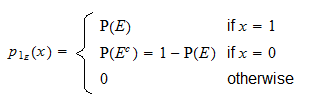## Properties

Indicator functions enjoy the following properties.

### Powers

The-th power ofis equal to:Proof

This is a consequence of the facts thatcan be eitheror, and### Expected value

The expected value ofis equal toProof

The proof is as follows: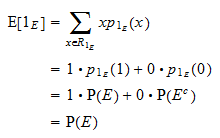### Variance

The variance ofis equal toProof

Thanks to the usual variance formula and the powers property above, we obtain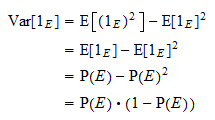### Intersections

Ifandare two events, thenProof

If, thenand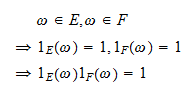If, thenand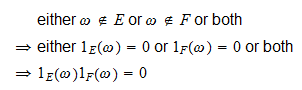### Indicators of zero-probability events

Letbe a zero-probability event andan integrable random variable. Then,Proof

While a rigorous proof of this fact is beyond the scope of this introductory exposition, this property should be intuitive. The random variableis equal to zero for all the sample points, except possibly for the points. The expected value is a weighted average of the valuescan take on, where each value is weighted by its respective probability. The non-zero valuescan take on are weighted by zero probabilities, somust be zero.

## Very similar concepts

In probability theory and statistics, there are two important concepts that are almost identical to that of an indicator variable:

1. the dummy variable.

## Solved exercises

Below you can find some exercises with explained solutions.

### Exercise 1

Consider a random variableand another random variabledefined as a function of.Expressusing the indicator functions of the eventsand.

Solution

Denote bythe indicator of the eventand denote bythe indicator of the event. We can writeas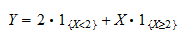### Exercise 2

Letbe a positive random variable, that is, a random variable that can take on only positive values.

Letbe a constant.

Prove thatwhereis the indicator of the event.

Solution

First note that the sum of the indicatorsandis always equal to:As a consequence, we can write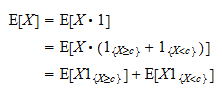Now, note thatis a positive random variable and that the expected value of a positive random variable is positive:Thus,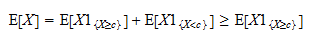### Exercise 3

Letbe an event and denote its indicator function by.

Letbe the complement ofand denote its indicator function by.

Can you expressas a function of?

Solution

The sum of the two indicators is always equal to:Therefore,